#istayhome

# The Pivot element and the Simplex method calculations

### Basic concepts and principles

The basis of the simplex algorithm is that there is not need to calculate the inverse of matrix B to calculate the extreme points of feasible region (Remember: B is an square submatrix of A with rank m).

Instead, a series of calculations lead to an element of matrix A called "Pivot element" and it is used to pass through other simple calculations, from one extreme point to the next one, testing each of them the optimality criterion, until one of these four cases holds .

### Simplex Algorithm and two-phase Method possible Outputs

1) The optimality criterion is fulfilled in one extreme point, in this case finite optimal solution holds. Example here

2) The optimality criterion holds, but there are infinite points also holds, this is the case with infinite solutions. Example here

3) Unbounded Solution: when the objective function can grow whatever we want within the feasible region. Example here

4) We can not continue iterating and not reached the criterion of optimality. At this case constraints are inconsistent and the problem has no solution Example here.
As it was said, to perform the calculations, in practice it is not necessary to invert the B matrix (remember that B is a square submatrix A of mxm and rank m), we create a table, called Tabeau for each iteration of the algorithm in which the calculations are much easier to do than to explain

The Simplex Tableau is constructed as follows:
 Base x1 ... xi ... xm
 Xb β1 ... βi ... βm
 Cb Cb1 ... Cbi ... Cbm
 a1 ... ak ... an y11 ... y1k ... y1n ... ... ... ... ... yi1 ... yik ... yin ... ... ... ... ... ym1 ... ymk ... ymn z1-c1 ... zk-ck ... zn-cn
In red we've left the pivot element that we will explain below

In this Simplex tableau, if there is an mxm identity submatrix of A, the vector Xb would be an extreme point that allows us to begin the iterations of the algorithm, is for him to calculate the zj - cj
Once calculated, we check ifon the optimality criterion hold, ie we test

zj - cj <0, ∀j

In case of non-compliance, we take the k index of j's that makes

zk - ck = max(zj - cj), such us zj - cj >0

ie, we take the highest positive in zj - cj.
Now, with this index k, we find the called the pivot element. To do this, divide each coordinate i of the vector Xb by its coordinate column vector i yik and calculate the minimal of positive, ie we calculate

yik = min {i: βi/yik, yik>0 }, being βi coordinates of vector Xb

If the previous minimum was -1 (there not exist positive), would be in the case of unbounded solution, as this would indicate that for every index i

βi/yik ≤ 0

and we would be in the case of unbounded solution as we have seen in Section Simplex Algorithm.

Otherwise we can find, as the index that minimizes yik. This is the number called Pivot Element

### Pivot Element of the Simplex Algorithm

It is called the Pivot element on Simplex Algoritm Tableau to those yik of the constraints matrix wich indexes i and k makes
k = max(zj - cj), such us zj - cj >0
i = min {i: βi/y ik, y ik>0 }

at this case, we have k, that will be the new index of x that enters the base i is the index Xb leaving the base.
Now recalculate the whole tableau in this new basis, for this we calculate the matrix A as follows

### Constraints matrix at new basis calculation

y'sj = ysj - (ysk * ysj)/yik
y'ij = yij/yik

This is the basis of the algorithm to invert the constraints matrix .

That is, for the pivot row, the new coefficients are calculated by dividing by the pivot, and for the other rows are calculated by subtracting the ai coefficient in the pivot row multiplied by the coefficient corresponding to the column divided by the pivot element.

We will see the end of this section a practical example of the algorithm.

### Extended theory

We have the following problems:
1) How to find a first solution to starts the iterations? This is equivalent to finding an identity mxm submatrix of A

2) In the event that the problem contains inequality constraints P. How to perform the operations?

3) Some of the variables may not be restricted in sign. How to operate in this case?

4) We have inconsistency or redundancy in operation. What to do in this case?

5) What if we want to maximize and not minimize?

1) The idea is to find a submatrix B of A, such that, by rearranging the columns if necessary B is the mxm identity, in this case the b vector, is a solution to our problem by taking as vectors those whose columns form the matrix B
In the event that there is identity matrix B, we use the two-phase method.

2) We would for any row i of matrix AIn this case we define a slack variable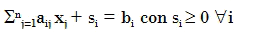And if we have to row i, we define the slack variableSo, our problem with inequalities, leads to an equalities issue.

3) If for any component i, the variable is not restricted in sign, then we can to do

xi = xi1 - xi2 con xi1, xi2 ≥0

4) If we have incompatibility, we can try to reformulate the problem so that such constraints be consistent. Incompatible constraits is the same as saying that our problem can not be resolved
At redundancy case. we can simply discard the redundant constraints.

5) If we have as an objective function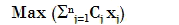We can reformulate the problem so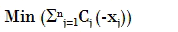Thus, we have the rule that "maximize one variable is the same as that variable change signs and minimize that variable".

Example

Max(- x1 + 3x2)
-x1 + 2x2 ≤6
x1 + x2 ≤5
2x1 + 2x2 ≤10

Aplicamos el punto 3) for constraints 2 and 3, both are the same, we remove one of them, so we

Max(- x1 + 3x2)
-x1 + 2x2 ≤6
x1 + x2 ≤5

We apply the point 5), ie 'maximize a variable is the same as the sign change of variable and minimize the variable'.

Min(x1 - 3x2)
-x1 + 2x2 ≤6
x1 + x2 ≤5

We apply the point 2)

Min(x1 - 3x2 + 0x3 + 0x4)
-x1 + 2x2 + x3 = 6
x1 + x2 + x4 = 5

Now we have solved the point 1),because we already have an identity submatrix of A, the variables concerning to x3, x4 variables
We build the Simplex Tableau and solve the problem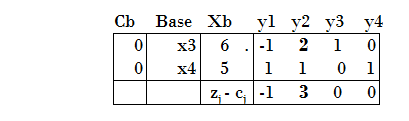We take the minimum of the positive of zj - cj
In our case 3, is given for the variable y2, so enters at the basis and the index 2, (or k=2).
Now we calculate the rate that leaves the base, for this we divide each element of the vector Xbk by its corresponding in the column k of matrix a, ie, the minimum of 6/2 and 5/1     min (6/2, 5/1) = 3 , the index is out of the base 3 and the pivot element is 2
We can perform one iteration of the simplex algorithm, is thus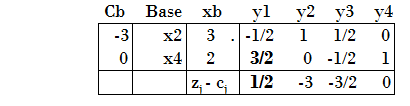Let's see how we have performed these calculations:
1)For the pivot row, we have simply divided each coefficient by the Pivot element.
2) For the other rows we have subtracted for each one the coefficient of Pivot column multiplied by the coefficient at the Pivot row and divided by the Pivot, for example

3/2 = 1 - (-1) * 1/2 = 1 + 1/2
0 = 1 - 2 * 1/2 = 1 - 1
-1/2 = 0 - 1 * 1/2 = 0 - 1/2
1 = 1 - 1 * 0/1 = 1 - 0

3) And the same for the Xb, simply 2 = 5 - 1 * 6/2 = 5 - 3

We perform now another iteration, now the leaving out index is 4 and the entering index is 1, so we haveNow fulfills the criterion on optimality because
zj - cj > 0, ∀j
Thus we have a finite optimal solution
x1 = 4/3
x2 = 11/3
What makes an optimization objective function
s = -4/3 + 33/3 = 29/3
In summary, the simplex algorithm forwards from one extreme point to another by pivoting the constraint matrix is, by a basis change and calculating the coefficients in the new basis by the pivot element.

# Was useful? want add anything?

Post here

### bashir:

2013-12-13 10:00:56
thanks... It was useful for me...

Post here